# Excel Mid function and Midb usage(6 examples, include with Len and find, variable length)

Lionsure 2019-09-23 Original by the website

The Mid function is used to extract the specified number of characters from the specified position from the text, and the MidB function is used to extract the specified number of bytes from the specified position from the text in excel; the difference is: the former counts each character, whether single-byte or double-byte, as 1, no matter what the default language setting is; and the latter counts each double-byte character as 2 when you have enabled the editing of a language that supports DBCS and then set it as the default language, Otherwise, it counts each character as 1.

The Mid function and the MidB function are often combined with functions such as Len, LenB, Find, VlookUp, LookUp, and Match; Mid is combined with Len and LenB to implement reverse extraction of characters, and Len and LenB are usually used to calculate the starting position or the length of strings that are extracted; Mid is combined with Find to extract the unit, and Find is usually used to determine the starting position of the character to be extracted.

### I, Excel Mid function and MidB function syntax

1. Mid function expression: MID(Text,Start_Num,Num_Chars)

2. MidB function expression: MIDB(Text,Start_Num,Num_Bytes)

3. Description:

A. Mid function counts full-width(such as Chinese characters, Japanese characters, Korean characters) and half-width characters(letters or numbers) as a character, and the MidB function counts the full-width as two bytes and half-width as one byte.

B. Start_Num is the starting position of the character to be extracted, if the first character is to be extracted, then Start_Num is 1; Start_Num must be greater than or equal to 1, otherwise it will return the value error #VALUE!; if Start_Num is greater than the length of text, it will return a null value (ie ""); if Start_Num is less than the length of text but is greater than length of text after adding Num_Chars, only returns the characters up to the end of text.

C. Num_Chars is to extract the number of characters, it must be greater than or equal to 0, otherwise it will return the value error #VALUE!.

D. Num_Bytes is the number of bytes to extract characters, it must be greater than 0, otherwise it will return the wrong value #VALUE!.

### II, The examples of Excel Mid function

(I) Extract from the first character and return a null value("")

1. If you want to extract "Excel" from the "Excel2016 Tutorial"; select the cell B1, enter the formula =MID(A1,1,5), press Enter, return to "Excel"; double-click the cell B1 and change the 1 to 19 in the formula, press Enter to return to the null value; the operation steps are as shown in Figure 1: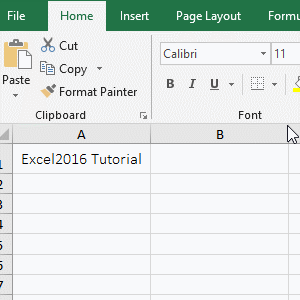Figure 1

2. Formula description:

A. Formula =MID(A1,1,5), A1 is the text to extract the character, 1 is the Start_Num of the character to be extracted, and 5 is the Num_Chars. The formula means that takes 5 characters from the first character from the "Excel2016 tutorial", that is, extracting Excel.

B. Formula =MID(A1,19,5) To extract from the 19th character of the text in A1("Excel2016 Tutorial"), since the text has only 18 characters (the space is also a character), the null value is returned(which is "").

(II) return only to the last character of the text

1. If you want to extract the "Tutorial" in the "Excel2016 Tutorial". Double-click the cell B1, copy the formula =MID(A1,11,9) to B1, press Enter, return to the word "Tutorial"; the operation steps are as shown in Figure 2: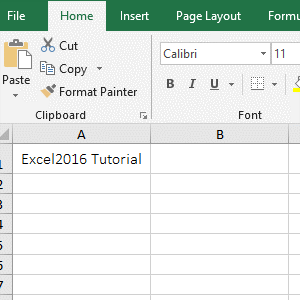Figure 2

2. The 10th character is a space, the 11th character is "T" in the "Excel2016 Tutorial", it has only 7 letters after it, and the formula =MID(A1,11,9) is to extract 9 characters, due to from the letter "T" to the end is less than 9 characters, so only the last character is extracted.

Hint: If the characters to be extracted are long, do not know how many characters to extract, set the third argument to a value larger than the number of characters to be extracted.

(III) The number of extracted characters is less than 0, return #VALUE! Error

1. Double-click the cell B1, copy the formula =MID(A1,6,1) to B1, press Enter, return 2; double-click B1, change 1 to 0, press Enter, return to empty; double-click B1 again, 0 is changed to -1, press Enter, return value error #VALUE!; operation process steps, as shown in Figure 3: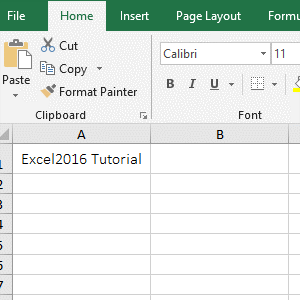Figure 3

2. The formula =MID(A1,6,1) means to return 1 character from the 6th character, that is, return 2; formula =MID(A1,6,0) returns 0 characters, that is, returns null; formula =MID(A1,6,-1) returns -1 characters, since the number of characters to be extracted must be greater than 0, the return value is incorrect #VALUE!.

### III, The examples of Excel MidB function

(I) Extracting numbers and letters

1. If you want to extract "Excel2016" from the "Excel2016 Tutorial". Double-click the cell B1, copy the formula =MIDB(A1,1,9) to B1, press Enter, return to "Excel2016"; the operation steps are as shown in Figure 4: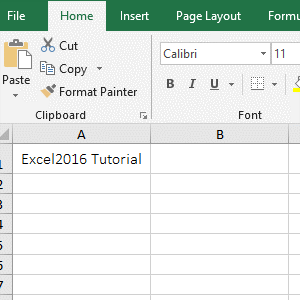Figure 4

2. A1 is the text to extract the characters, 1 is the Start_Num, 9 is the Num_Bytes in the formula =MIDB(A1,1,9); the formula means that extract 9 characters from the first position E of the string "Excel2016 Tutorial", which happens to be "Excel2016", indicates that the MidB function is the same as the Mid function, treating both numbers and letters as one byte.

### IV, The application example of Excel Mid function and MidB function

(I) The combination of Mid + Len functions to extract characters in reverse(Excel formula mid from right to left)

1. If you want to start from the right side, extract the 8 characters from "Excel2016 Function Usage Tutorial". Double-click the cell B1, copy the formula =MID(A1,LEN(A1)-8+1,8) to B1, press Enter, return to "Tutorial"; the operation steps are as shown in Figure 5: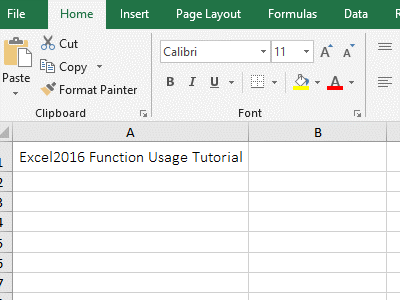Figure 5

2. Formula =MID(A1,LEN(A1)-8+1,8) explanation:

A. the 8 in the formula is the number of characters to be extracted; LEN(A1) is used to calculate the number of characters in A1, and the full-width and the half-width are counted as one character. The return result is 33, and LEN(A1)-8+1 is equal to 26, that is, the Start_Num of the character to be extracted is calculated, and the Start_Num is exactly the letter "T".

B. The formula becomes =MID(A1,26,8), and then 8 characters are extracted from the 26th character, that is, the "Tutorial" is obtained.

Hint: The MidB function can also extract characters in reverse. The formula =MID(A1,LEN(A1)-8+1,8) can be written in MidB: =MIDB(A1,LEN(A1) -8 +1,8), copy the formula to B2, press Enter, also return to the "Tutorial", the operation process steps, as shown in Figure 6: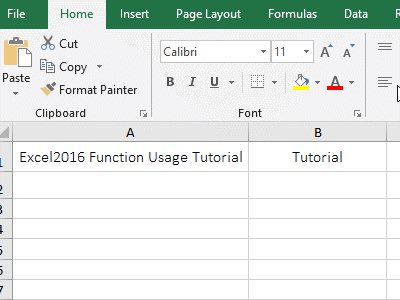Figure 6

(II) Excel Mid Find variable length(Mid + Find function combination to start from any specified character)

1. If you want to extract the unit after the number in column A. Double-click the cell B1, copy the formula =MID(A1,FIND("l",A1),3) to B1, press Enter, return to "l/b"; select B1 and move the mouse to the cell fill handle in the bottom right corner of B1, after the mouse becomes the bold black plus, hold down the left button and double-click to extract the units of the remaining cells; the operation steps are as shown in Figure 7: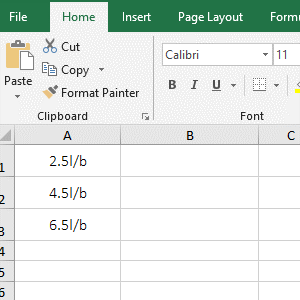Figure 7

2. Formula =MID(A1,FIND("l",A1),3) description:

A. FIND("l",A1) is used to return the index of the "l" in the text of A1, "l" is the text to be searched, the text int A1 is the text to find "l", in addition, FIND("l",A1) also omits the last argument Start_Num, the default search starts from the first character. FIND("l",A1) returns 4.

B. The formula becomes =MID(A1,4,3), that is, 3 characters are intercepted from the 4th character, and the result is "l/b"(liter/bottle).